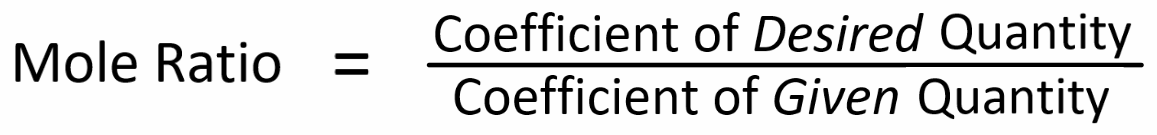# All Topics     |   Breslyn.org   |     Stoichiometry Topics

### Solving Limiting Reagent Problems

Often in chemical reactions we'll have different amounts of each chemical reacting. For example, with a campfire we'll run out of wood before we run out of oxygen in the air. The wood limits the chemical reaction. It is called the limiting reagent. To solve these problems we use the same skills used in equation stoichimetry.

They only new skill is determining which chemical we run out of first in the reaction. Video from Bozeman Science.

### Key Ideas:

There are two approaches to finding the limiting reagent:
• comparing moles of each reactant.

• calculating and comparing how much of each product will be produced for each reactant.

Both approaches involve using the mole ratio.

More Help and Practice:

### Thinking Tools

It is helpful to visualize two mole maps connected by the mole ratio to solve stoichiometry problems:One way to find the the mole ratio is:We get the coefficients for the mole ratio from the balanced equation.

Printable Mole Map

Go To Top
Subscribe